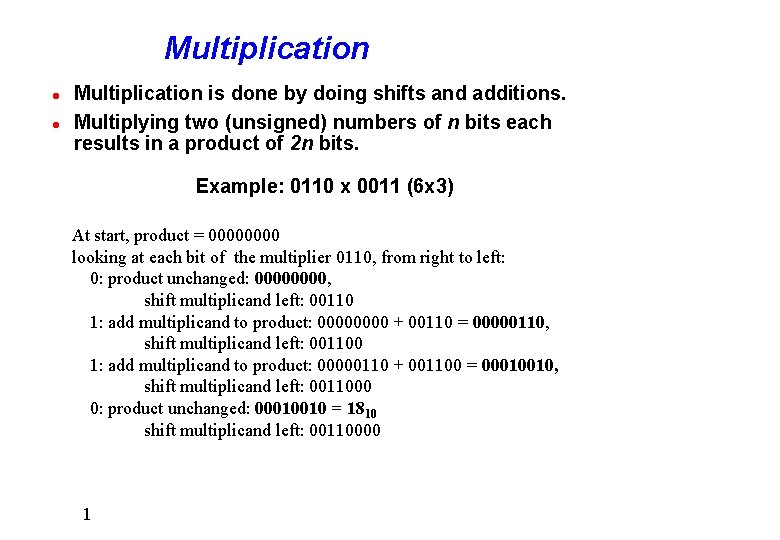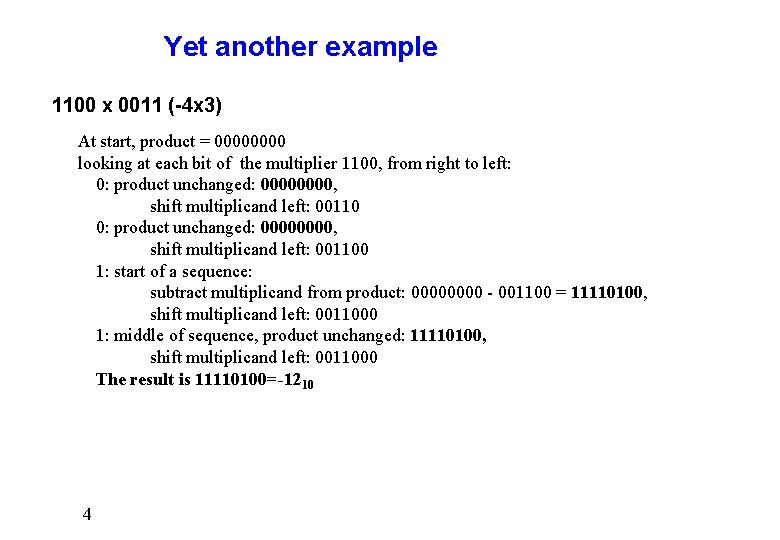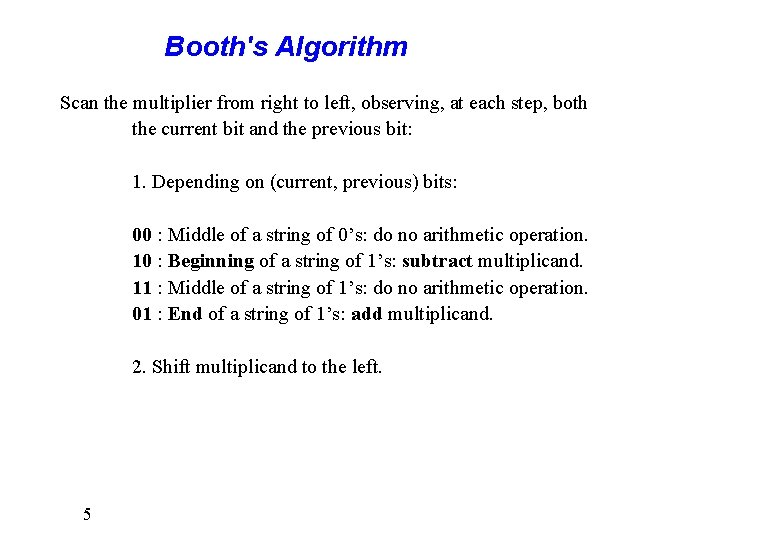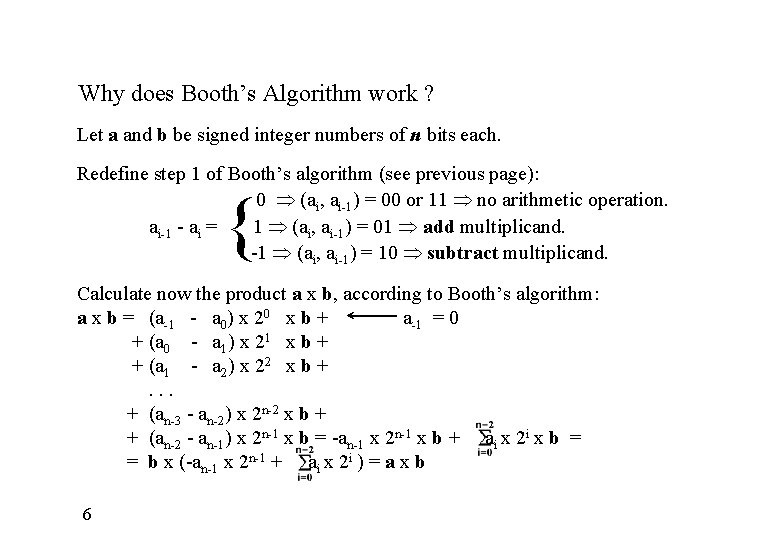# Multiplication l l Multiplication is done by doing

• Slides: 6Multiplication l l Multiplication is done by doing shifts and additions. Multiplying two (unsigned) numbers of n bits each results in a product of 2 n bits. Example: 0110 x 0011 (6 x 3) At start, product = 0000 looking at each bit of the multiplier 0110, from right to left: 0: product unchanged: 0000, shift multiplicand left: 00110 1: add multiplicand to product: 0000 + 00110 = 00000110, shift multiplicand left: 001100 1: add multiplicand to product: 00000110 + 001100 = 00010010, shift multiplicand left: 0011000 0: product unchanged: 00010010 = 1810 shift multiplicand left: 00110000 1Multiplying Singed Numbers Main difficulty arises when signed numbers are involved. • Naive approach: convert both operands to positive numbers, multiply, then calculate separately the sign of the product and convert if necessary. • A better approach: Booth’s Algorithm. Booth’s idea: if during the scan of the multiplier, we observe a sequence of 1’s, we can replace it first by subtracting the multiplicand (instead of adding it to the product) and later, add the multiplicand, after seeing the last 1 of the sequence. 2Example 0110 x 0011 (6 x 3) This can be done by (8 -2)x 3 as well, or (1000 - 0010) x 0011 (using 8 bit words) At start, product = 0000 looking at each bit of the multiplier 0110, from right to left: 0: product unchanged: 0000, shift multiplicand left: 00110 1: start of a sequence: subtract multiplicand from product: 0000 - 00110 = 11111010, shift multiplicand left: 001100 1: middle of sequence, product unchanged: 11111010, shift multiplicand left: 0011000 0: end of sequence: add multiplicand to product: 11111010 + 0011000 = 00010010 = 1810 shift multiplicand left: 00110000 3Yet another example 1100 x 0011 (-4 x 3) At start, product = 0000 looking at each bit of the multiplier 1100, from right to left: 0: product unchanged: 00000000, shift multiplicand left: 001100 1: start of a sequence: subtract multiplicand from product: 0000 - 001100 = 11110100, shift multiplicand left: 0011000 1: middle of sequence, product unchanged: 11110100, shift multiplicand left: 0011000 The result is 11110100=-1210 4Booth's Algorithm Scan the multiplier from right to left, observing, at each step, both the current bit and the previous bit: 1. Depending on (current, previous) bits: 00 : Middle of a string of 0’s: do no arithmetic operation. 10 : Beginning of a string of 1’s: subtract multiplicand. 11 : Middle of a string of 1’s: do no arithmetic operation. 01 : End of a string of 1’s: add multiplicand. 2. Shift multiplicand to the left. 5Why does Booth’s Algorithm work ? Let a and b be signed integer numbers of n bits each. Redefine step 1 of Booth’s algorithm (see previous page): 0 (ai, ai-1) = 00 or 11 no arithmetic operation. ai-1 - ai = 1 (ai, ai-1) = 01 add multiplicand. -1 (ai, ai-1) = 10 subtract multiplicand. { Calculate now the product a x b, according to Booth’s algorithm: a x b = (a-1 - a 0) x 20 x b + a-1 = 0 + (a 0 - a 1) x 21 x b + + (a 1 - a 2) x 22 x b +. . . + (an-3 - an-2) x 2 n-2 x b + + (an-2 - an-1) x 2 n-1 x b = -an-1 x 2 n-1 x b + ai x 2 i x b = = b x (-an-1 x 2 n-1 + ai x 2 i ) = a x b 6